8. 查找（Search）## 8.0. 何为查找？

101 张三 键盘清洁与维修
102 李四 母猪产后护理与保养
103 李四 计算机开关机专业操作员

• 如果找到了，即查找成功，返回元素的信息；

• 如果找遍所有元素还没找到，说明这群元素中没有符合要求的元素，即查找失败，返回一个可以明显标记失败的值，比如“空记录”或“空指针”。

## 8.1. 顺序查找（Sequential Search）

• 若某个关键字和目标值相等，则查找成功，返回所查元素的信息；
• 若没有一个关键字和目标值相等，则查找失败，返回失败标识值。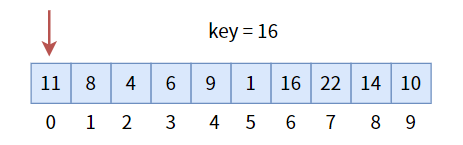``````/**
* @description: 从头到尾遍历整个数组，查找目标值 key，返回其下标 index
* @param {int} *array 数组 为了说明问题简单，这里的数组元素不重复
* @param {int} length 数组长度
* @param {int} key 目标值
* @return {int} 如果找到，返回目标值下标；否则返回 -1
*/
int sequential_search(int *array, int length, int key)
{
for (int index = 0; index < length; index++) {
if (array[index] == key) {
return index;
}
}
return -1;
}``````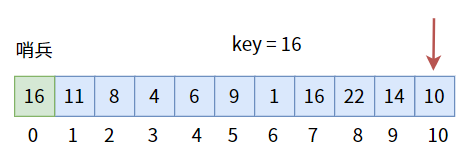``````/**
* @description: 顺序查找改进，增加哨兵
* @param {int} *array array 不存放数据元素，充当哨兵
* @param {int} length 数组长度
* @param {int} key 目标值
* @return {int} 返回0，即哨兵下标，则查找失败；否则成功
*/
int sequential_search_pro(int *array, int length, int key)
{
array = key; // 哨兵
int index = length - 1;
while (array[index] != key) {
index--;
}
return index;
}``````

## 8.2. 二分查找（Binary Search）

• 如果相等，则查找成功，返回中间元素的信息；
• 如果不相等，说明目标值 `key` 在左半区或右半区：
• 若目标值 `key`小于中间元素的关键字，则来到左半区；
• 若目标值 `key` 大于中间元素的关键字，则来到右半区；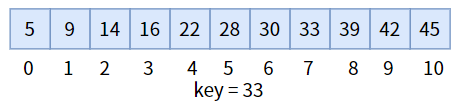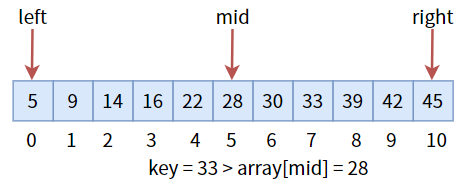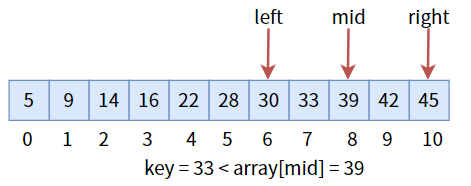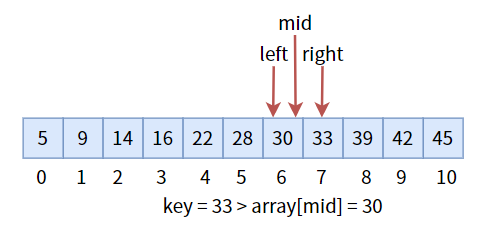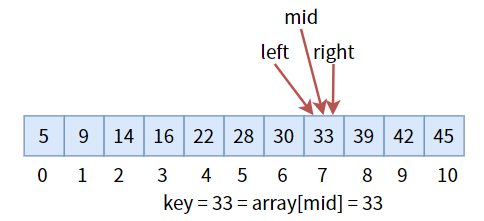``````/**
* @description: 二分查找
* @param {int} *array 有序数组
* @param {int} length 数组长度
* @param {int} key 目标值，和关键字比较
* @return {int} 返回目标值下标；若查找失败，则返回 -1
*/
int binary_search(int *array, int length, int key)
{
int left, mid, right;
left = 0;
right = length - 1;
while (left <= right) {
mid = (left + right) / 2; // 中间下标
if (key < array[mid]) { // key 比中间值小
right = mid - 1; // 更新最右下标，进入左半区
} else if (key > array[mid]) { // key 比中间值大
left = mid + 1; // 更新最左下标，进入右半区
} else {
return mid; // key 等于中间值，返回其下标
}
}
return -1; //未找到，返回 -1
}``````

## 8.3. 二叉查找树（Binary Search Tree）

### 8.3.0. 是什么？

• 若左子树不为空，则左子树的所有结点值皆小于根结点值
• 若右子树不为空，则右子树的所有结点值皆大于根结点值
• 左右子树也是二叉排序树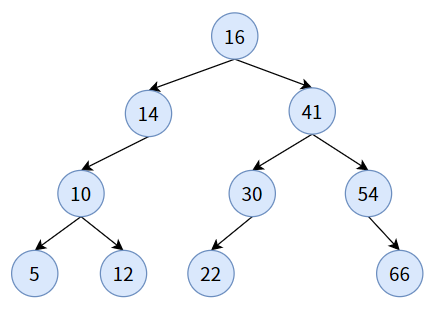### 8.3.1. 查找

``````int bst_search(BSTNode *root, int key, BSTNode **p, BSTNode *q)
{
if (root == NULL) { // 空树，查找失败
*p = q;
return 0;
}
if (key == root->data) { // 目标值相等根结点的值，查找成功
*p = root;
return 1;
} else if (key < root->data) { // 目标值小于根结点的值，递归进入左子树
return bst_search(root->left_child, key, p, root);
} else { // 目标值大于根结点的值，递归进入右子树
return bst_search(root->right_child, key, p, root);
}
}``````

• 指针 `root` 始终指向根结点， 标识了与目标值比较的结点；

• 二级指针 `p` 指向最终查找的结果。如果查找成功，则指向“指向‘找到的结点’的指针”；如果查找失败，则指向“指向‘上次最后一个访问的结点’的指针”。

• 指针 `q` 初始为空，以后始终指向上一个根结点。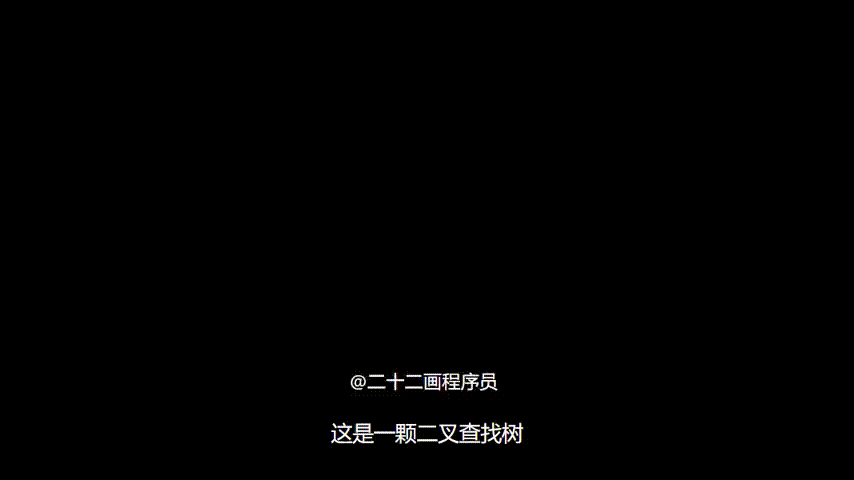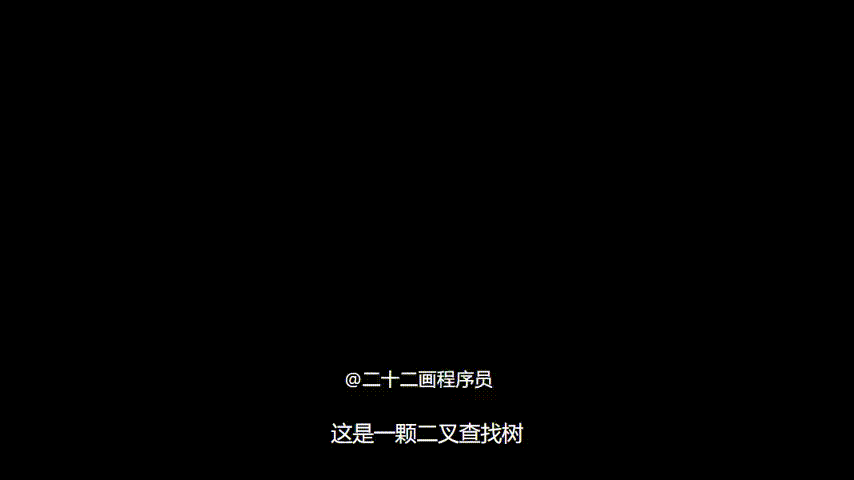1. 都是有序的。二分查找是全部有序，二叉排序数是局部有序。
2. 都是将一组数划分成若干区域。二分查找通过三个标志位，二叉排序树通过树结构。
3. 都是通过比较目标值和“区域分界点”的大小，从而确定目标值在哪个区域中。在二分查找中与中间标志位比较，在二叉查找树中与根结点比较。

### 8.3.2. 创建和插入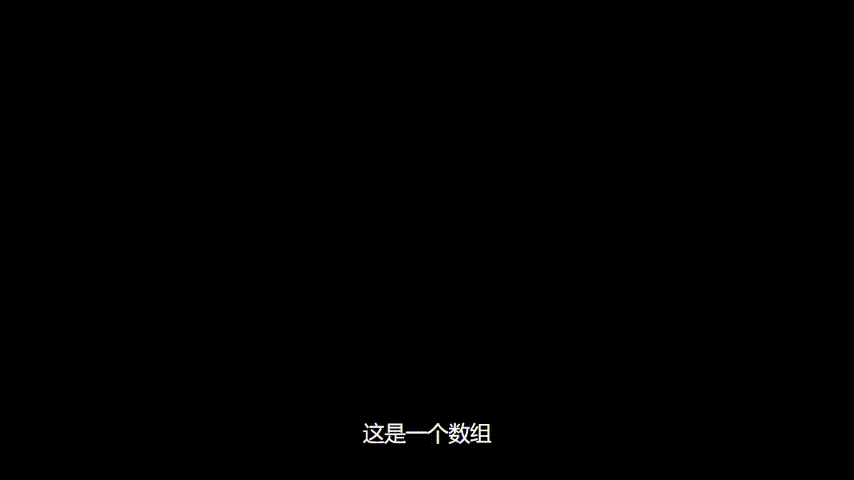``````int insert_bst_node(BSTNode **p_root, int key)
{
BSTNode *p;
if (bst_search(*p_root, key, &p, NULL) == 0) { // 没有查找到 key
BSTNode *new = create_bst_node(key); // 创建新结点
if (p == NULL) { // 空树，新节点直接作为根结点
*p_root = new;
return 1;
}
if (key < p->data) { // 插入值小于 p 的值，插入到左子树
p->left_child = new;
return 1;
}
if (key > p->data) {  // 插入值大于 p 的值，插入到右子树
p->right_child = new;
return 1;
}
}
return 0; // 插入失败
}``````

``````void create_bst(BSTNode **root, int *array, int length)
{
// 循环向空二叉查找树中插入若干结点
for (int i = 0; i < length; i++) {
insert_bst_node(root, array[i]);
}
}``````

### 8.3.3. 删除

【情况一】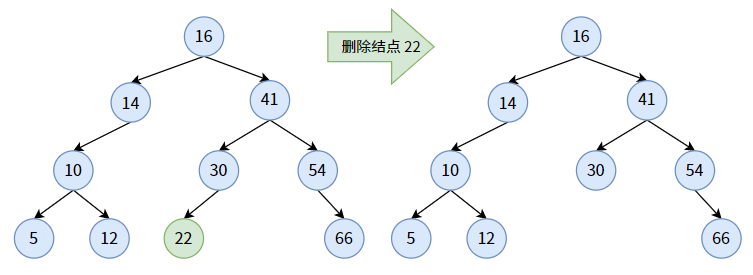【情况二】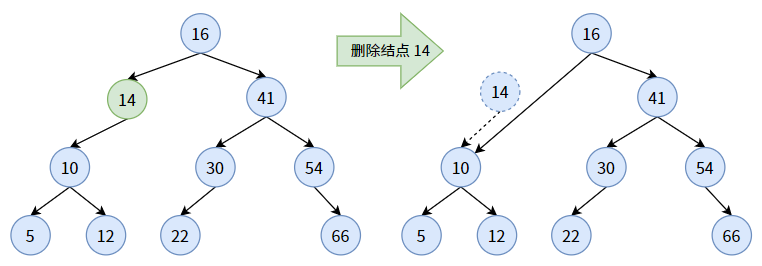【情况三】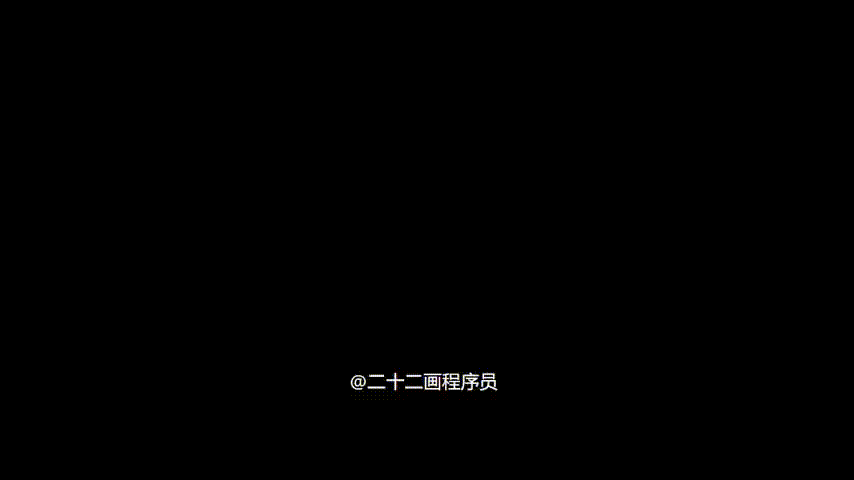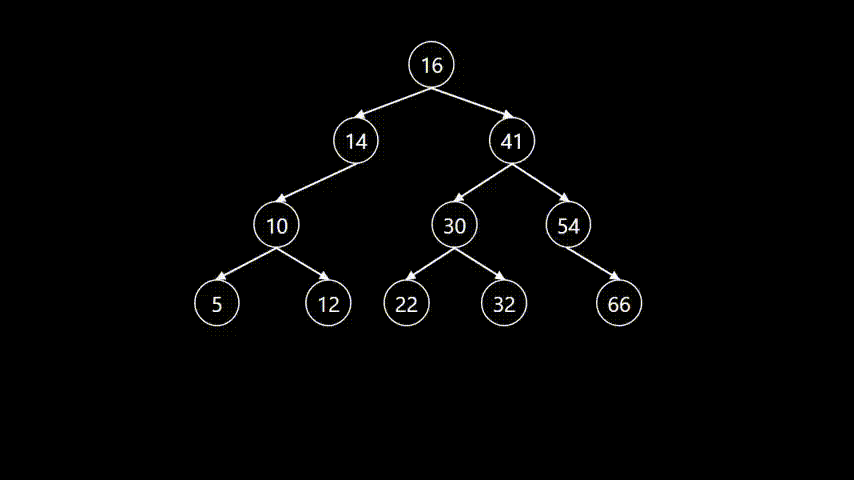``````/**
* @description: 删除目标值结点
* @param {BSTNode**} 二级指针，指向 指向树根结点的指针 的指针
* @param {int} key 目标值
* @return {int} 删除成功返回1；否则返回0
*/
int delete_bst_node(BSTNode **p_root, int key)
{
if (*p_root == NULL) {
return 0;
}
if (key == (*p_root)->data) { // 找到要删除的目标值 key
rebuild_bst_after_delete(p_root);
} else if (key < (*p_root)->data) { // 目标值小于根结点，递归进入左子树
return delete_bst_node(&(*p_root)->left_child, key);
} else { // 目标值大于右子树，递归进入右子树
return delete_bst_node(&(*p_root)->right_child, key);
}
}``````

``````/**
* @description: 删除某个结点后重新调整二叉查找树
* @param {BSTNode**} p_node 指向待删除结点的二级指针
* @return {int} 成功返回1；失败返回0
*/
int rebuild_bst_after_delete(BSTNode **p_node)
{
BSTNode *prev, *tmp;
tmp = *p_node;
if ((*p_node)->left_child == NULL) { // 左子树为空，重接右子树
*p_node = (*p_node)->right_child;
free(tmp);
return 1;
}
if ((*p_node)->right_child == NULL) { // 右子树为空，重接左子树
*p_node = (*p_node)->left_child;
free(tmp);
return 1;
}
// 左右子树均不为空
if ((*p_node)->left_child != NULL && (*p_node)->right_child != NULL) {
prev = (*p_node)->left_child;
while (prev->right_child != NULL) { // 找到待删除结点的直接前驱
tmp = prev;
prev = prev->right_child;
}
(*p_node)->data = prev->data; // 赋值
if (tmp != *p_node) { // 待删除结点有子孙结点
tmp->right_child = prev->left_child;
} else { // 待删除结点只有两个孩子结点，没有子孙结点
tmp->left_child = prev->left_child;
}
free(prev);
return 1;
}
return 0;
}``````22
Lv1
……

16

6

3